Disclosure: This post may contain affiliate links, meaning when you click the links and make a purchase, we receive a commission.

# VLOOKUP Formula to Compare Two Columns in Different Sheets!

If you have two big columns and you want to compare them, you can use Excel’s VLOOKUP function. In this article, I will show how you can use VLOOKUP formula to compare two columns in different sheets.

We have two worksheets (SSA and Mongabay) as below.

In the SSA worksheet, you will find the 100 most popular given names for male babies born during the last 100 years, from the year 1918 to 2017 (source).

And in the Mongabay worksheet, you will find the 100 most common male names in the U.S. (source)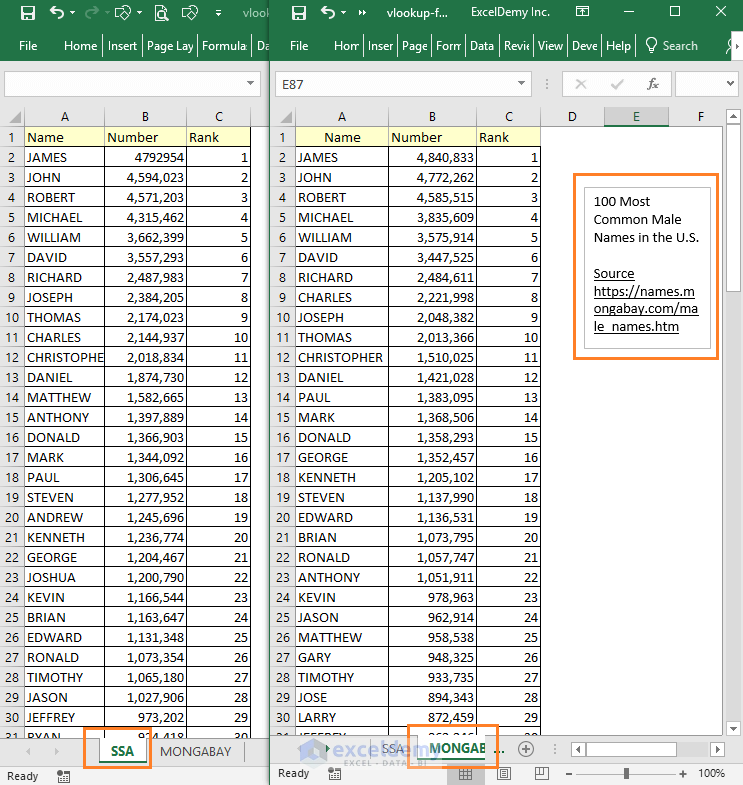We shall compare these two worksheets using Excel VLOOKUP formula.

Let’s compare the SSA worksheet names with the names of Mongabay worksheet.

## Using VLOOKUP Formula to Compare Two Columns in Different Worksheets

1) Add a new column (Comparing with Mongabay) after the Rank column in the SSA worksheet. And then input this formula in cell D2: =VLOOKUP(A2, mongabay_data, 1, FALSE)

mongabay_data = MONGABAY!\$A\$2:\$C\$101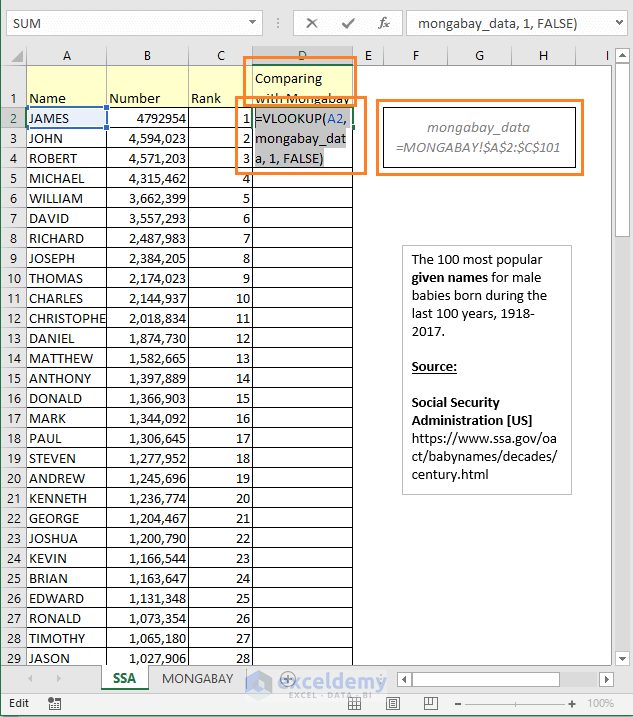Input this formula in D2: =VLOOKUP(A2, mongabay_data, 1, FALSE)

2) Press Enter. You will see James name is showing in the cell. James is showing in the cell because James name is found in the Mongabay worksheet. Now double-click on the Fill Handle icon on the bottom left corner of the cell D2.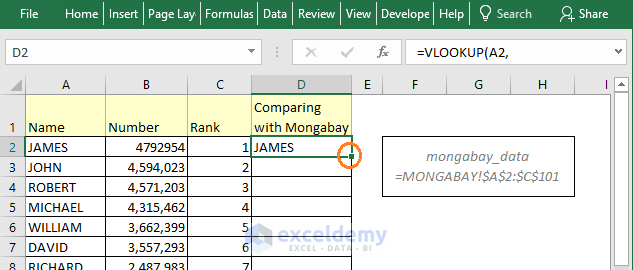Double click on the Fill Handle to fill all the cells with the formula of cell D2.

3) The formula of cell D2 will be copied down to all the cells in the column.

We get the first mismatch in the D32 cell. The formula here is showing #N/A error. So, the formula did not find a match for the name Jacob.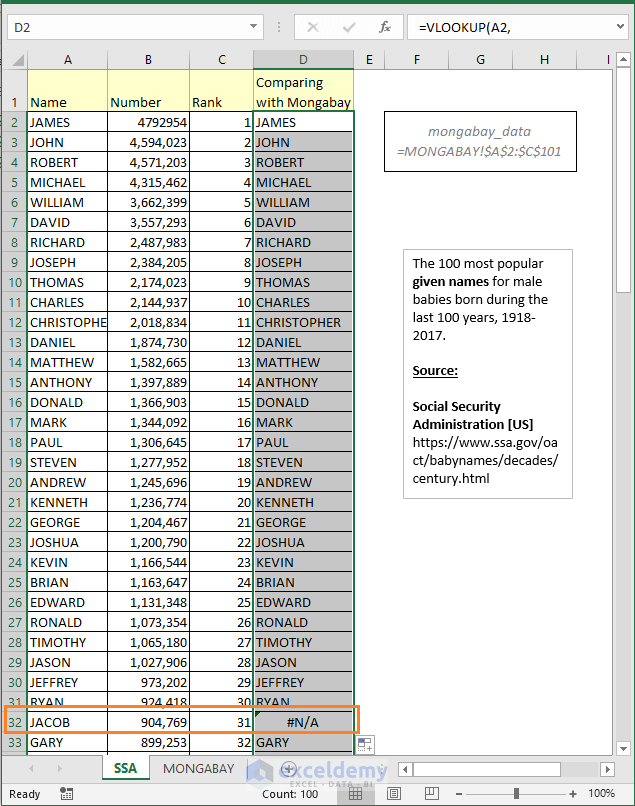At cell D32, we get the first #N/A error value. So, Jacob name is not matching.

4) More mismatches are here. So, these names (Alexander, Tyler, Nathan, Zachary, Kyle) are losing their zeal in recent time.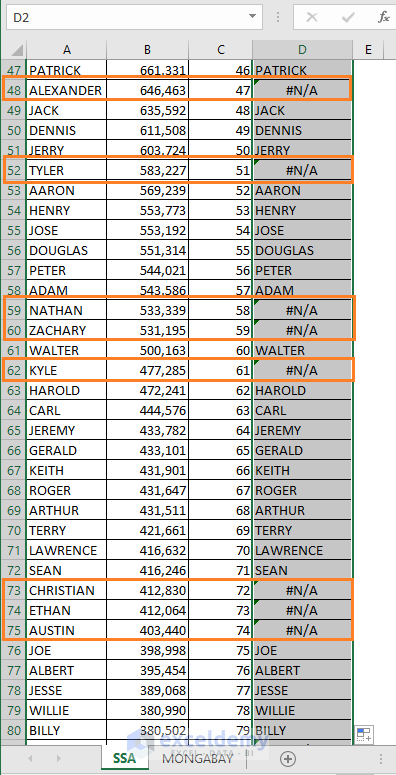Some more errors.

This is a very straightforward VLOOKUP formula. Here is a brief of Excel VLOOKUP function. If you need a revision of this function, pass some time on the following image.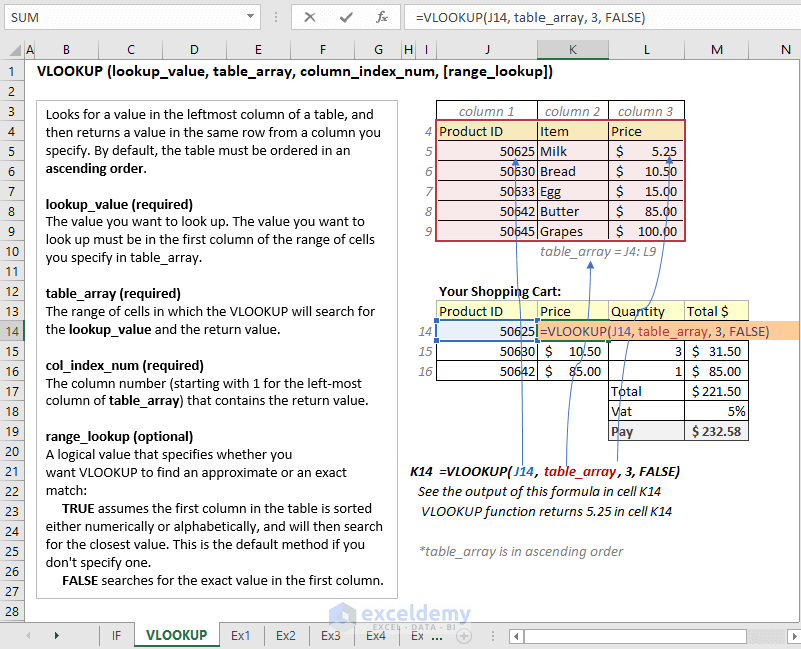Excel VLOOKUP Function.

## IFERROR and VLOOKUP combo to treat the #N/A error

This time, let’s use this formula in cell D2 and then copy it to other cells of the column.

`D2=IFERROR(VLOOKUP(A2,mongabay_data,1,FALSE),"--[Not Found]")`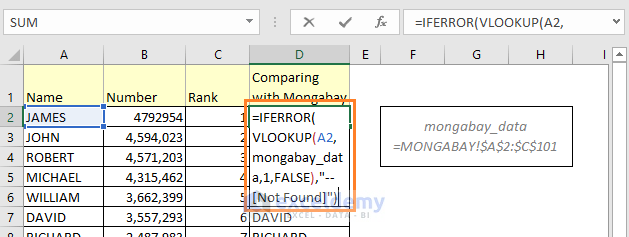Use this formula in cell D2: `=IFERROR(VLOOKUP(A2,mongabay_data,1,FALSE),"--[Not Found]")`

You see, this time cell D32 is not showing the error value. Rather than it is showing this text `"- -[Not Found]"` value.

### How does this formula work?

To understand this formula, you have to be familiarized with the IFERROR excel function.

The syntax of IFERROR function: =IFERROR(value, value_if_error)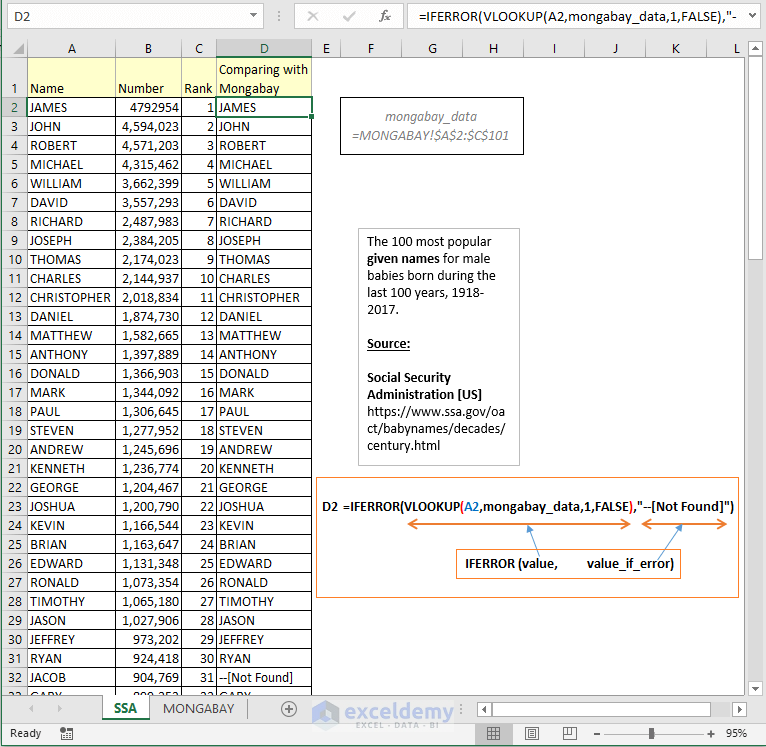Decoding the formula.

Let’s see how the above formula works

• As the value of IFERROR function, we have input our VLOOKUP formula. So, if there is no error, the output of the VLOOKUP formula will be the output of the IFERROR function.
• As the value_if_error argument, we have passed this value, “- -[Not Found]”. So, if IFERROR function finds an error in the cell, it will output this text, “- -[Not Found]”.

## IF, ISNA, and VLOOKUP combo to Handle the #N/A error

We can also use IF, ISNA and VLOOKUP combo to handle that #N/A errors.

This time in cell D2, build this formula: `=IF(ISNA(VLOOKUP(A2,mongabay_data,1,FALSE)),"Not Matched", "Matched")`

And then use this formula for other cells in the column.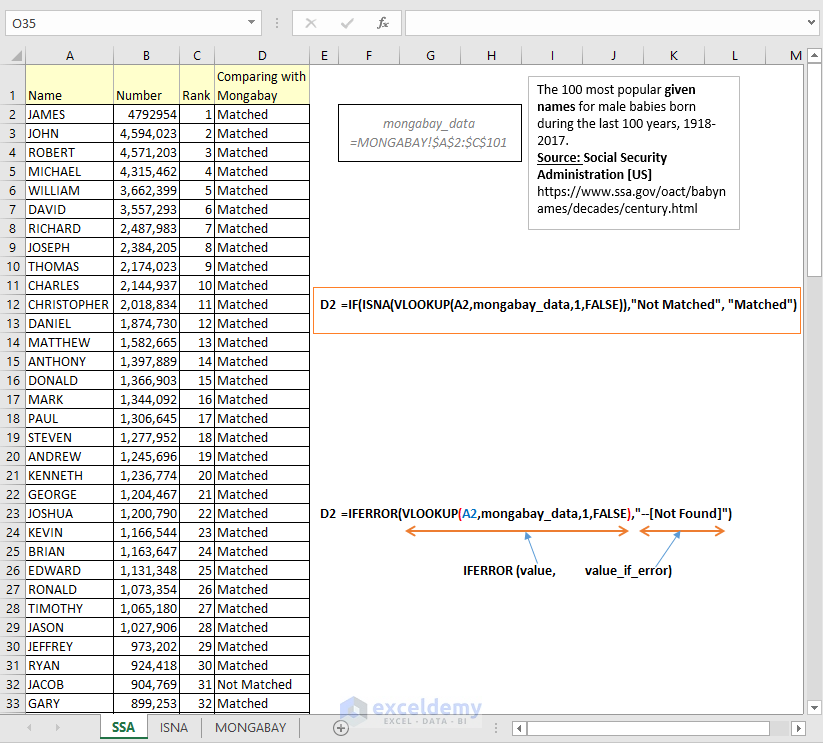Use this formula in cell D2: `=IF(ISNA(VLOOKUP(A2,mongabay_data,1,FALSE)),"Not Matched", "Matched")`

### How does this formula work?

To understand this formula, you have to know how IF and ISNA functions work.

Here is the syntax of IF function: =IF(logical_test, value_if_true, value_if_false)

Take a look at the following image to see how the IF function works.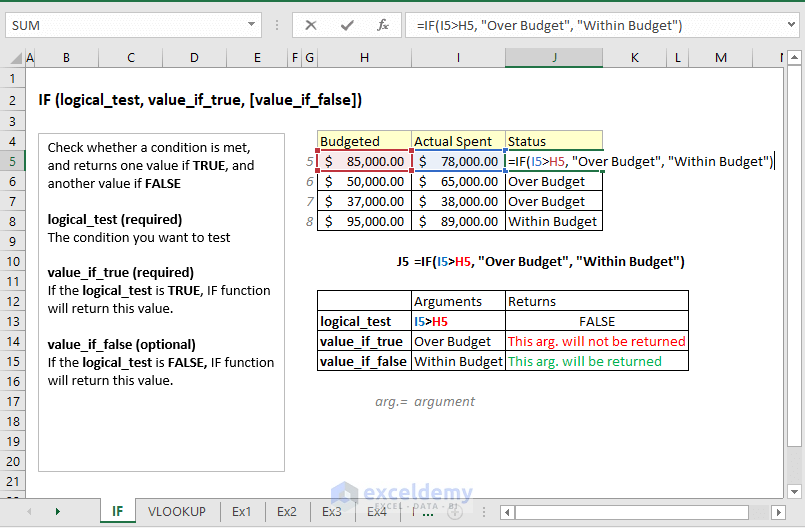Excel IF Function.

Here is the syntax of ISNA function: =ISNA(value)

And this is how ISNA function works.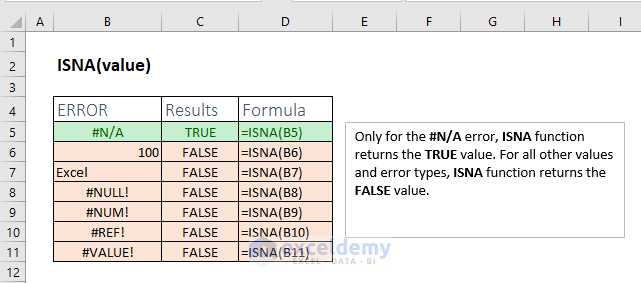ISNA Excel Function

Let’s now see how the following formula works.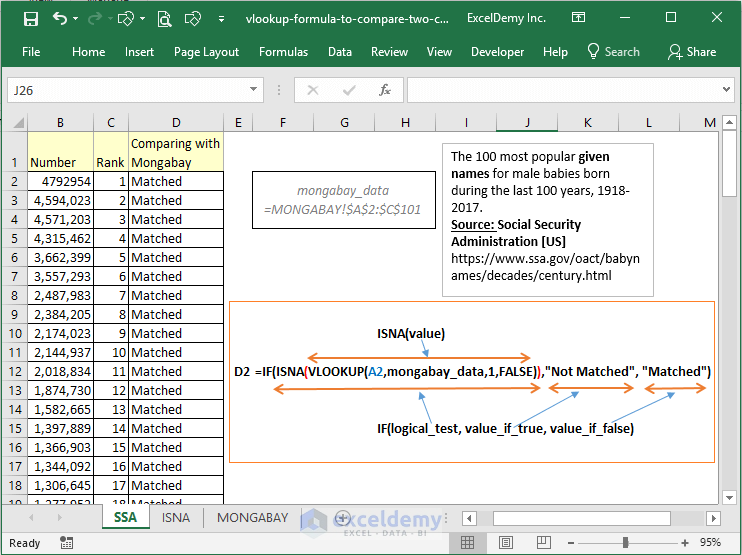Decoding Excel formula

• As the logical_test argument of IF function, we have passed the ISNA function and ISNA function holds our VLOOKUP If the VLOOKUP formula returns a #N/A error, ISNA function will return the TRUE value. When the logical_test is true IF function will return this value: “Not Matched”.
• If the VLOOKUP formula returns a value (no error), ISNA function will return FALSE So, IF function’s logical_test argument will be False. When logical_test is False IF function will return this value: “Matched”.

So, these are the VLOOKUP formulas that you can use to compare two columns in different Excel worksheets. Hope this helps.

Happy Excelling!

Hello!
Welcome to my Excel blog! It took me some time to be a fan of Excel. But now I am a die-hard fan of MS Excel. I learn new ways of doing things with Excel and share here. Not only how to guide on Excel, but you will get also topics on Finance, Statistics, Data Analysis, and BI. Stay tuned!

1. Reply• Reply